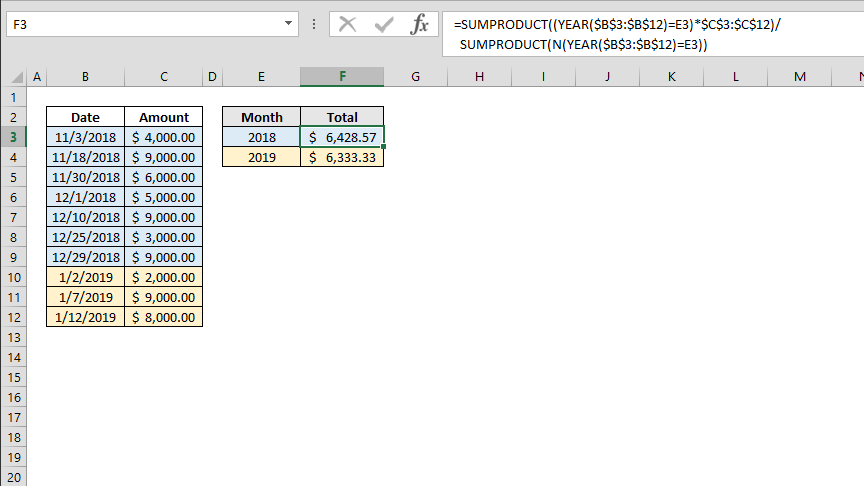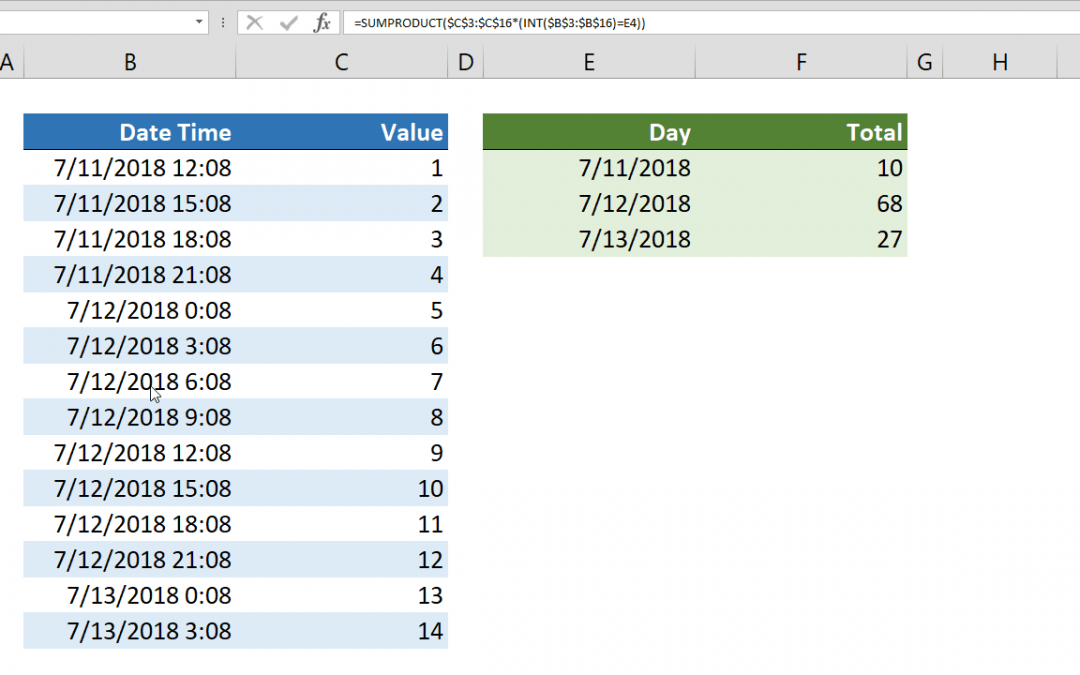## How to do a cell count if not equal to a variable or even an error code

You can use the COUNTIFS function to count the values in ranges that meet certain criteria. The criteria can contain a not equal logic as well. However, the COUNTIFS function requires individual arguments for each range-criteria pair. This requirement can make it...## What does double minus -- do in Excel

The double minus, also known as double unary, is an operation to convert Boolean values into ones and zeroes. Simply; TRUE to one (1) and FALSE to zero (0). A unary operation is an operation with only one operand, which is in contrast to binary operations that use two...## How to create a year-by-year average calculator

Although the AVERAGEIF and AVERAGEIFS function can sum values by a given condition, they won’t work if you want to use the output in other functions. In this article, we’re going to show you how to create a year-by-year average calculator with help of the...## How to sum by date in Excel without time

Excel already has functions like the SUMIF and the SUMIFS for summing data by groups. However; they can't work if you have date-time values combined. This article shows How to sum by date in Excel without time using SUMPRODUCT and INT functions. Syntax =SUMPRODUCT(...